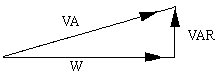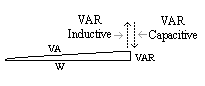PowerFactor-EnergyConservation
Motor Power Factor and Energy Conservation

Energy conservation is a hot topic these days and everybody "knows" that low power factor can mean waste of electrical energy. Many questions are asked about the power factor of induction motors. Some competitors stress the high power factor of their motors. Why don't we?

Induction motors are only one of the kinds of electrical equipment that tend to reduce a plant's power factor. And it's the overall plant system's power factor that counts. There are ways of correcting a low system power factor, so maximum motor power factor isn't vital. System power factor correction is often the better way.

A Quick Review of the Theory

True Power, measured in watts (W), is the power, drawn by the electrical resistance of a system, that does useful work.

Reactive Power, measured in volt-amperes, reactive, (VAR) is the power stored in and discharged by the inductive motors, transformers and solenoids all draw reactive power.

Apparent Power, measured in volt-amperes (VA) is the voltage on an AC system multiplied by all the current that flows in it. It is the vector sum of the true and the reactive power.

Power Factor, is the ratio of the true power used in a system to the apparent power drawn from the source. It's usually expressed in percent: W/VA x 100. The cosine of the angle between VA and W in this vector diagram ø is a measure of power factor. The larger the VAR current the lower the power factor.Only the true power (W) in a system does useful work. But the utility has to generate and distribute what really flows: The apparent power (VA). Utilities usually only meter watts, but a utility can measure a plant's overall power factor and it's common to charge a rate penalty if that power factor is low. Within the plant, the power distribution system also has to be sized to distribute and switch the apparent power, not just useful watts. In short, the difference between useful power and apparent power, as indicated by power factor, represents power that does no work but costs the user money.

Why Not High Motor Power Factor?

As the diagram indicates, if the VAR vector is short, the power factor will be high. So it might seem that motors with high power factor will help, because they will contribute less to the overall system VAR vector. But... First: Motor load may not have much effect on system power factor. This is true when:

1. Motor load is relatively small in comparison to resistance load (W) on the plant system, drawn by such equipment as plant lighting and resistance heating.
2. Most of the induction motor load is represented by large, high-speed motors. Their power factor is inherently high, and the power factor of the fewer small motors won't mean much.
3. The plant uses some synchronous motors. These don't increase the VAR vector shown in the diagram, they tend to decrease it.
4. Motors are only part of the inductive load responsible for the length of the diagram's VAR vector. Almost any plant has some power transformers probably welding transformers, possible solenoid-operated mechanisms, and induction heating equipment. Also there is "stray inductance", in the plant's wiring and in theory these wiring systems are pure resistance loads.
5. High motor power factor is wasted if the motor is oversized for the driven load, or runs much of the time at reduced load.

In the above situations a high motor power factor won't affect overall system power factor much. Probably not enough to justify the cost and other disadvantages of motors designed for maximum power factor.

Second: You don't get as good a motor design by concentrating on high power factor. The motor designer has to consider a number of parameters such as temperature rise, torque characteristics and efficiency, as well as power factor, and he can't optimize them all. It's costly to try to design both high power factor and high efficiency into a motor, and some of the design changes that improve power factor, such as a reduced air gap, actually have the opposite effect on efficiency.

The Better Way

No matter what motors do to a plant system power factor, it can be corrected, and that's the better way. The VAR vector in the diagram above represents inductive reactance. But there's also capacitive reactance, which produces an opposite VAR vector. If a system is being affected by both kinds of reactance, they tend to cancel each other. In the system vector diagram below, capacitive VAR's are almost as great as inductive VAR's so W more nearly equals VA, and W/VA x 100 -- the system power factor -- is high.How:

Sometimes rotating capacitors are used to put capacitive reactance into a plant system, but static capacitors are more common. Maybe one central bank to correct the entire plant system, or a bank at each several plant load centers. Or, an individual capacitor installation at each motor. Which of these approaches is best for a given plant requires analysis of many variables, and we are not in a position to make general recommendations. A customer in doubt should contact the major capacitor manufacturers for detailed information and expert advice.

To Sum Up

Now you see why we don't go the highest-power-factor routed in motor design. Efficiency and other desirable characteristics have to be designed into the motor, because there's no good way -- external to the motor -- to get their effect. But the effect of motor power factor on plant system power factor, to whatever extent it has an effect, can be compensated for by external means and capacitors can improve power factor to 95-98%, a greater improvement than can be designed into a motor. External correction has the big advantage of correcting for the transformers and other equipment on the plant system that also tend to lower the power factor. With capacitors, a facility's system power factor can be "tailored" to match a utility's billing structure, so that the facility pays only for the degree power factor corrections that will really save money.